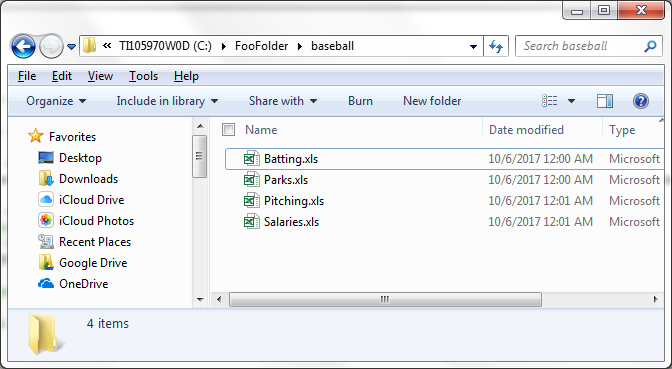Search

# Importing Multiple Excel Files into Access As Separate Table

Ken Snell has created a series of Access macros which can be used to import Excel files. He has posted them to this site. One of these is named, 'Import Data from All Worksheets in All EXCEL Files in a single Folder into Separate Tables via TransferSpreadsheet (VBA)'. I've posted it below with the beginning Sub and End Sub lines necessary for it to function in Visual Basic.

Sub ImportExcels()

Dim blnHasFieldNames As Boolean, blnEXCEL As Boolean, blnReadOnly As Boolean Dim intWorkbookCounter As Integer Dim lngCount As Long Dim objExcel As Object, objWorkbook As Object Dim colWorksheets As Collection Dim strPath As String, strFile As String Dim strPassword As String

' Establish an EXCEL application object On Error Resume Next Set objExcel = GetObject(, "Excel.Application") If Err.Number <> 0 Then Set objExcel = CreateObject("Excel.Application") blnEXCEL = True End If Err.Clear On Error GoTo 0

' Change this next line to True if the first row in EXCEL worksheet ' has field names blnHasFieldNames = False

' Replace C:\MyFolder\ with the actual path to the folder that holds the EXCEL files strPath = "C:\MyFolder\"

strFile = Dir(strPath & "*.xls")

intWorkbookCounter = 0

Do While strFile <> ""

intWorkbookCounter = intWorkbookCounter + 1

Set colWorksheets = New Collection

Set objWorkbook = objExcel.Workbooks.Open(strPath & strFile, , _ blnReadOnly, , strPassword) For lngCount = 1 To objWorkbook.Worksheets.Count colWorksheets.Add objWorkbook.Worksheets(lngCount).Name Next lngCount

' Close the EXCEL file without saving the file, and clean up the EXCEL objects objWorkbook.Close False Set objWorkbook = Nothing

' Import the data from each worksheet into a separate table For lngCount = colWorksheets.Count To 1 Step -1 DoCmd.TransferSpreadsheet acImport, acSpreadsheetTypeExcel9, _ "tbl" & colWorksheets(lngCount) & intWorkbookCounter, _ strPath & strFile, blnHasFieldNames, _ colWorksheets(lngCount) & "\$" Next lngCount

' Delete the collection Set colWorksheets = Nothing ' Uncomment out the next code step if you want to delete the ' EXCEL file after it's been imported ' Kill strPath & strFile

strFile = Dir()

Loop

If blnEXCEL = True Then objExcel.Quit Set objExcel = Nothing

End Sub

Note especially that on the line beginning 'blnHasFieldNames =' the setting should be 'True' if the Excel files you're importing have column headings, and on the line beginning 'strPath =' you should specify the path to the folder containing the Excel files you want to import.A macro like this comes in really useful when you're importing dozens of Excel files, but in this example we're working with just four files.The macro will quickly create tables like these.